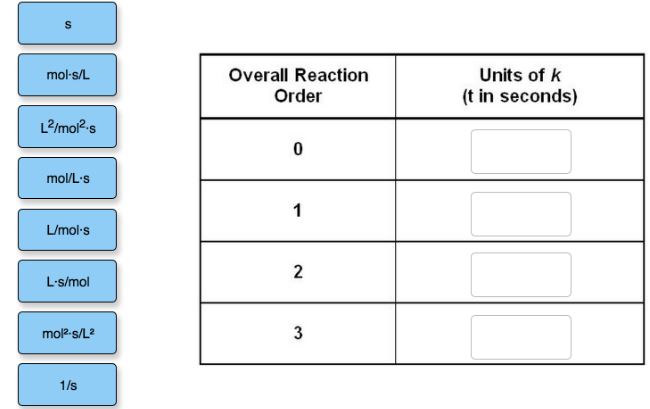Problem: Fill in the units for the apparent rate constant for each reaction order given below.

FREE Expert Solution
97% (389 ratings)
Problem Details

Fill in the units for the apparent rate constant for each reaction order given below.What scientific concept do you need to know in order to solve this problem?

Our tutors have indicated that to solve this problem you will need to apply the Rate Law concept. You can view video lessons to learn Rate Law Or if you need more Rate Law practice, you can also practice Rate Law practice problems .

What is the difficulty of this problem?

Our tutors rated the difficulty of Fill in the units for the apparent rate constant for each re... as medium difficulty.

How long does this problem take to solve?

Our expert Chemistry tutor, Dasha took 4 minutes to solve this problem. You can follow their steps in the video explanation above.

What professor is this problem relevant for?

Based on our data, we think this problem is relevant for Professor Li & Thompson's class at PURDUE.